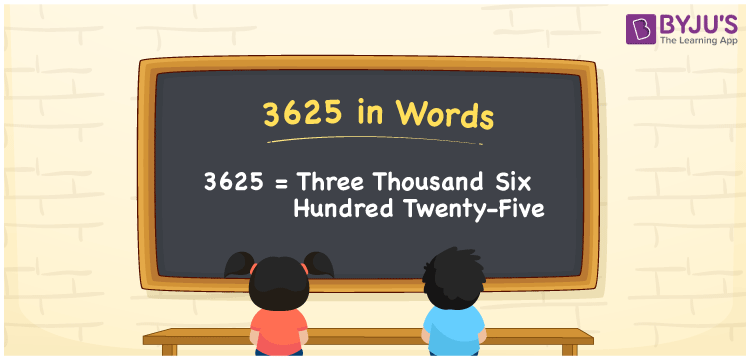# 3625 in words

3625 in words is written as Three Thousand Six Hundred and Twenty Five. 3625 represents the count or value. The article on Counting Numbers can give you an idea about count or counting. The number 3625 is used in expressions that relate to money, distance, length, year and others. Let us consider an example for 3625. ”I owe you Three Thousand Six Hundred and Twenty Five rupees”.

 3625 in words Three Thousand Six Hundred and Twenty Five Three Thousand Six Hundred and Twenty Five in Numbers 3625

## 3625 in English Words## How to Write 3625 in Words?

We can convert 3625 to words using a place value chart. The number 3625 has 4 digits, so let’s make a chart that shows the place value up to 4 digits.

 Thousands Hundreds Tens Ones 3 6 2 5

Thus, we can write the expanded form as:

3 × Thousand + 6 × Hundred + 2 × Ten + 5 × One

= 3 × 1000 + 6 × 100 + 2 × 10 + 5 × 1

= 3625

= Three Thousand Six Hundred and Twenty Five.

3625 is the natural number that is succeeded by 3624 and preceded by 3626.

3625 in words – Three Thousand Six Hundred and Twenty Five.

Is 3625 an odd number? – Yes.

Is 3625 an even number? – No.

Is 3625 a perfect square number? – No.

Is 3625 a perfect cube number? – No.

Is 3625 a prime number? – No.

Is 3625 a composite number? – Yes.

## Solved Example

1. Write the number 3625 in expanded form

Solution: 3 × 1000 + 6 × 100 + 2 × 10 + 5 × 1

We can write 3625 = 3000 + 600 + 20 + 5

= 3 × 1000 + 6 × 100 + 2 × 10 + 5 × 1

## Frequently Asked Questions on 3625 in words

Q1

### How to write 3625 in words?

3625 in words is written as Three Thousand Six Hundred and Twenty Five.
Q2

### Is 3625 a perfect square number?

No. 3625 is not a perfect square number.
Q3

### Is 3625 a prime number?

No. 3625 is not a prime number.